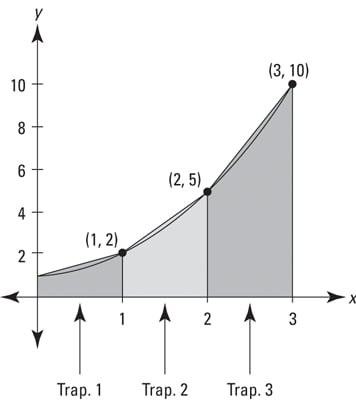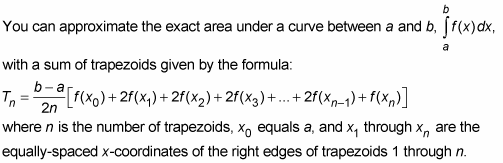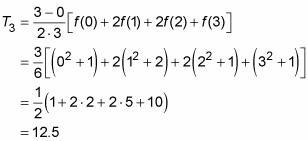##### Trigonometry Workbook For DummiesWith the trapezoid rule, instead of approximating area by using rectangles (as you do with the left, right, and midpoint rectangle methods), you approximate area with — can you guess? — trapezoids.

Because of the way trapezoids hug the curve, they give you a much better area estimate than either left or right rectangles. And it turns out that a trapezoid approximation is the average of the left rectangle and right rectangle approximations. Can you see why? (Hint: The area of each trapezoid is the average of the areas of the two corresponding rectangles in the left and right rectangle sums.)

The figure below shows three trapezoids drawn under the function x2 + 1.From the look of this figure, you might expect a trapezoid approximation to be better than a midpoint rectangle estimate, but in fact, as a general rule, midpoint sums are about twice as good as trapezoid estimates.

If you’ve already worked out the left and right rectangle approximations for a particular function and a certain number of rectangles, you can just average them to get the corresponding trapezoid estimate (for this problem, you know the answer you’re going to get is (8 + 17)/2 = 12.5). If not, here’s the formula:

The Trapezoid Rule:For the function in the above figure with three trapezoids, here’s the math:Even though the formal definition of the definite integral is based on the sum of an infinite number of rectangles, you might want to think of integration as the limit of the trapezoid rule at infinity. The further you zoom in on a curve, the straighter it gets. When you use a greater and greater number of trapezoids and then zoom in on where the trapezoids touch the curve, the tops of the trapezoids get closer and closer to the curve. If you zoom in “infinitely,” the tops of the “infinitely many” trapezoids become the curve and, thus, the sum of their areas gives you the exact area under the curve. This is a good way to think about why integration produces the exact area — and it makes sense conceptually — but it’s not actually done this way.